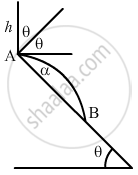Department of Pre-University Education, KarnatakaPUC Karnataka Science Class 11
Advertisement Remove all ads

# Solve the Previous Problem If the Coefficient of Restitution is E. Use θ = 45 ∘ E = 3 4 and H = 5 M . - Physics

Sum

Solve the previous problem if the coefficient of restitution is e. $\text{ Use } \theta = 45^\circ\, e = \frac{3}{4} \text{ and h = 5 m } .$

Advertisement Remove all ads

#### Solution

Given:
The angle of inclination of the plane, θ = 45°
A ball falls on the inclined plane from a height (h) of 5 m.

The coefficient of restitution,$e = \left( \frac{3}{4} \right)$
The velocity with which ball strikes the inclined plane is given as,
$v = \sqrt{2g \times 5} = 10 m/s$

The ball makes an angle β with the horizontal, after the collision.
The horizontal component of velocity, 10cos45° remains unchanged.
However, the velocity in perpendicular direction to the plane after the collision will now be:

$v_1 = e \times 10 \sin45^\circ$

$= \left( \frac{3}{4} \right) \times 10 \times \frac{1}{\sqrt{2}}$

$= (3 . 75) \sqrt{2} m/s$

=$\text{ Similarly,} v_2 = 5\sqrt{2}\text{m/s}$

$\text{ Now,}$

$u = \sqrt{v_2^2 + v_1^2}$

$= \sqrt{50 + 28 . 125}$

$= \sqrt{78 . 125}$

$= 8 . 83 \text{m/s}$Angle of reflection from the wall is given as,

$\beta = \tan^{- 1} \frac{(3 . 75\sqrt{2})}{5\sqrt{2}}$

$= \tan^{- 1} \left( \frac{3}{4} \right) = 37^\circ$
Angle of projection α = 90 − (θ + β)
$\Rightarrow$ α = 90° − (45° + 37°) = 8°
Let the distance where the ball falls after the collision be L.
⇒ x = L
cos θ
Angle of projection (α) = −8°
Now,
Y = $x \tan \alpha - \frac{g x^2 se c^2 \alpha}{2 u^2}$
$\Rightarrow$ −L sin θ = L cos θ × tan 8° $- \frac{g}{2}\frac{L^2 \cos^2 \theta \sec^2 80^\circ\ }{(u )^2}$

$\Rightarrow - \sin 45^\circ = \cos 45^\circ \times \tan 8^\circ - \frac{10 \cos^2 45 \sec^28^\circ }{(8 . 83 )^2}$
On solving the above equation, we get:
= 18.5 m

Is there an error in this question or solution?
Advertisement Remove all ads

#### APPEARS IN

HC Verma Class 11, 12 Concepts of Physics 1
Chapter 9 Centre of Mass, Linear Momentum, Collision
Q 45 | Page 162
Advertisement Remove all ads
Advertisement Remove all ads
Share
Notifications

View all notifications

Forgot password?